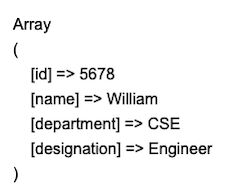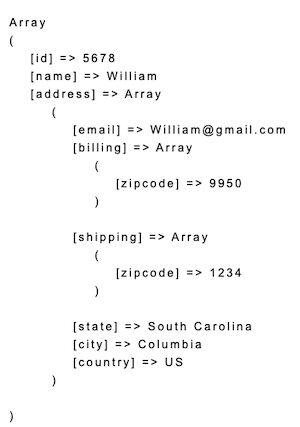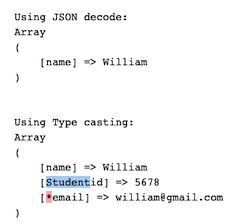# PHP Object to Array Convert using JSON Decode

The PHP object to array conversion makes it easy to access data from the object bundle. Most of the API outputs object as a response.

Some APIs may return a complex object structure. For example, a mixture of objects and arrays bundled with a response. At that time, the object to array conversion process will simplify the data parsing.

This quick example performs a PHP object to array conversion in a single step. It creates an object bundle and sets the properties.

It uses JSON encode() decode() function for the conversion. The json_decode() supplies boolean true to get the array output.

## Quick example

PHP object to array conversion in a line using json_decode

``````<?php
\$object = new StdClass();
\$object->id = 5678;
\$object->name = "William";
\$object->department = "CSE";
\$object->designation = "Engineer";

\$result = json_encode(\$object);
// converts object \$result to array
\$output = json_decode(\$result, true);

print "<pre>";
print_r(\$result);
?>
``````

#### Output

After decoding, the output array is printed to the browser. The below screenshot shows the output of this program.## Different ways of converting a PHP object to array

When converting an object to array, the object property ‘name:value’ pairs will form an associative array.

If an object contains unassigned properties then it will return an array with numerical keys.

There are two ways to achieve a PHP object to array conversion.

1. Typecasting object into an array.
2. Encoding and decoding object properties into an array of elements.

Typecasting is a straightforward method to convert the type of input data. The second method applies json_decode() on the given object. It supplied boolean true as a second parameter to get the output in an array format.

This article includes examples of using both of the above methods to perform the object to array conversion.

## PHP object to array using typecasting

This is an alternate method to convert an object type into an array. The below program uses the same input object.

It replaces the JSON encode decode via conversion with the typecasting statement. The output will be the same as we have seen above.

The PHP typecasting syntax is shown below. It prepends the target data type enclosed with parenthesis.

``````\$output = (target-data-type) \$input
``````

type-casting-to-convert-object-to-array.php

``````<?php
\$object = new StdClass();
\$object->id = 5678;
\$object->name = "William";
\$object->department = "CSE";
\$object->destination = "Engineer";

print"<pre>";
print_r( (array) \$object );
?>
``````

## Recursive object to array conversion

This example uses an input object with depth = 3. It adds more properties at a nested level at different depths. The hierarchical object bundle is set as the input for the conversion process.

This program defines a custom function to convert a PHP object to array. It performs the conversion recursively on each level of the input object.

converting-recursive-object-to-array.php

``````<?php
\$object = new StdClass();
\$object->id = 5678;
\$object->name = "William";

function objectToArray(\$object)
{
foreach (\$object as \$k => \$obj) {
if (is_object(\$obj)) {
\$object->\$k = objectToArray(\$obj);
} else {
\$object->\$k = \$obj;
}
}
return (array) \$object;
}

\$result = objectToArray(\$object);

print "<pre>";
print_r(\$result);
?>
``````

This is the output of the recursive PHP object to the array conversion program above.## Convert PHP class object into array

This example constructs a PHP class object bundle. The class constructor sets the properties of the object during the instantiation.

Then, the Student class instance is encoded to prepare object type data. The json_encode() function prepares the JSON object to supply it for decoding. The json_decode() converts the PHP object to array.

convert-class-object-into-array.php

``````<?php
class student
{
public function __construct(\$id, \$name, \$state, \$city, \$country)
{
\$this->id = \$id;
\$this->name = \$name;
\$this->state = \$state;
\$this->city = \$city;
\$this->country = \$country;
}
}

\$student = new student("5678", "William", "South Carolina", "Columbia", "US");
\$result = json_encode(\$student);
\$output = json_decode(\$result, true);
print "<pre>";
print_r(\$output);
?>
``````

## Check is_object() before conversion

It is good programming practice to check the data availability before processing. This example applies the is_object verification before converting a PHP object to an array.

This method verifies if the input is an object. PHP includes exclusive functions to verify data availability and its type. Example isset(), empty(), is_array() etc.

checking-object-before-conversion.php

``````<?php
class student
{

public function __construct(\$id, \$name, \$state, \$city, \$country)
{
\$this->id = \$id;
\$this->name = \$name;
\$this->state = \$state;
\$this->city = \$city;
\$this->country = \$country;
}
}
\$student= new student("5678", "William", "South Carolina", "Columbia", "US");

print "<pre>";
if (is_object(\$student)) {
echo "Input Object:" . '<br>';
\$result = json_encode(\$student);
print_r(\$result);
\$studentArray = json_decode(\$result, true);
}

if(!empty(\$studentArray) && is_array(\$studentArray)) {
echo "<br><br>Output Array:" . '<br>';
print_r(\$studentArray);
}
?>
``````

## Convert Private, Protected object of a class

The below program defines a class with private and protected properties. The PHP code instantiates the class and creates an object bundle.

It uses both the typecasting and decoding methods to convert the object into an array.

When using typecasting, the output array index of the private property contains the class name prefix. After conversion, the array index has a * prefix for the protected properties.

converting-private-protected-object.php

``````<?php
class Student
{
public \$name;
private \$id;
protected \$email;

public function __construct()
{
\$this->name ="William";
\$this->id = 5678;
\$this->email = "william@gmail.com";
}
}

print "<pre>";
\$student = new Student;
\$result = json_encode(\$student);
\$output1 = json_decode(\$result, true);
print "<br/>Using JSON decode:<br/>";
print_r(\$output1);

\$output2 = new Student;
print "<br/><br/>Using Type casting:<br/>";
print_r( (array) \$output2 );
?>
``````

This output screenshot shows the difference in the array index. Those are created from the private and protected properties of the class instance.## Accessing object properties with numeric keys

This code includes an associative array of student details. It also contains values with numeric keys.

When converting this array into an object, the associative array keys are used to access the object property values. There are exceptions to access properties if it doesn’t have a name.

The below code shows how to access objects with numeric keys. The key is enclosed by curly brackets to get the value.

problem-with-numerical-keys.php

``````<?php
\$inputArray = array(
'name' => 'William',
'email' => 'William@gmail.com',
'phone' => '12345678',
'REG5678'
);

\$student = (object) array(
'name' => 'William',
'email' => 'William@gmail.com',
'phone' => '12345678',
'REG5678'
);
echo '<pre>' . print_r(\$student, true) . '</pre>';
echo '<br />' . \$student->name;
echo '<br />' . \$student->email;
echo '<br />' . \$student->phone;
echo '<br />' . \$student->{0};
?>
``````

## Conclusion

We have seen the different ways of converting a PHP object to an array. The basic PHP typecasting has achieved an object conversion except for few special cases.

The PHP JSON encode decode process made the conversion with one line code. It accepts class objects and converts their properties into an array list.

The custom function processes recursive object to array conversion. It is to handle complex objects with mixed objects or arrays as its child elements.

## Comments to “PHP Object to Array Convert using JSON Decode”

• Zafer says:

Thanks

• Vincy says:

Welcome Zafer.

• Rob Salusbury says:

Hi Vincy,
Thank you for once again drastically simplifying what was previously mysterious. You have a rare gift for precision instruction, thank you so much for sharing with the world.
Best regards
Rob

• Vincy says:

Hi Rob,

Thank you so much for reading through and supporting Phppot all along.